# Building Bat Emergence Models with Twitter Data¶

## Justin Vincent (@justinvf)¶

### An algorithmshop.com production¶

This notebook will play with some ideas of using Twitter data to model information about bat emergence.

I went to the "bat bridge" twice while attending #scipy2014 in Austin, TX. There are over a million bats under that bridge, but I didn't get to see them come out en masse on either of our visits. Next time I'm in Austin, I'm hoping to go on nights where there is a high probability of bats swarming. My initial hypothesis is that wind and temperature have something to do with whether we get a swarm. If it's too windy, they don't want to fly up high and get blown about. If it's too hot (it's never too cold in Austin), then they are probably languid and will just order take-out that night. If the bats swarm, then people will probably tweet about the bats swarming, but that's not a given. No tweets could mean either no swarm, or that nobody felt like tweeting (must not be the SXSW week). So let's fake some data and then see what sort of parameters we can recover.

In :
from pymc import Bernoulli, Uniform, MCMC, Normal, Matplot, TruncatedNormal, deterministic, observed, poisson_like

from sklearn.metrics import f1_score
from sklearn.linear_model import LogisticRegression

import numpy as np
np.random.seed(45)

from matplotlib import pyplot as plt
%pylab inline

Populating the interactive namespace from numpy and matplotlib

In :
NUM_DAYS = 300

temperatures = np.random.normal(85, 7, NUM_DAYS)
temperatures /= temperatures.max()

wind_speeds = np.random.normal(0, 15, NUM_DAYS).clip(.2)
wind_speeds /= wind_speeds.max()

In :
plt.subplot(2,1,1)
plt.hist(temperatures);
plt.title('Temperatures')
plt.subplot(2,1,2)
plt.hist(wind_speeds)
plt.title('Wind speeds')
plt.tight_layout()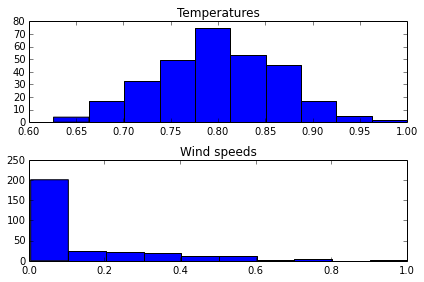In :
# In generating our fake data, we'll just make some random score involving wind speed and temp.
# The higher the grumpier!
grumpy_bat_score = temperatures * 3 + wind_speeds * 2

In :
plt.hist(grumpy_bat_score);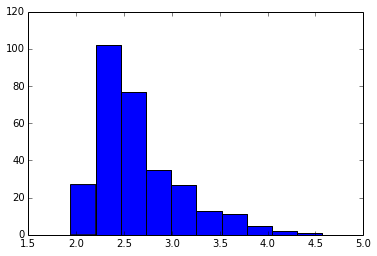Looking at that curve, I want to make the data so that if the score is below 2.4, you have a high chance of coming out and if it's above 3.2, you have a low chance. So lets make a sigmoid that does something like that.

In :
x = np.linspace(1.5, 4.5, 1000)
widget_a, widget_b = -5, 2.8
random_numbers = np.random.rand(NUM_DAYS)
bat_exit_days = None

def plot_sigmoid(a=-5, center=2.8):
global widget_a, widget_b, bat_exit_days
b = -center * a
widget_a, widget_b = a, b
values = 1 / (1 + np.exp(-(x*a + b)))
plt.plot(x, values)
plt.ylim([0,1])
plt.xlim([1.5,4.5])
bat_exit_probability = 1 / (1 + np.exp(-(grumpy_bat_score * a + b)))
bat_exit_days = random_numbers < bat_exit_probability
print("Bats swarmed out of the bridge {} days out of {} (a = {} b = {})".format(bat_exit_days.sum(), NUM_DAYS, a, b))


Play with the widget and make up a probability distribution that looks good to you. Higher magnitude values of a controll the sharpness of the sigmoid function. The center is the midpoint of the distribution.

In :
from IPython.html.widgets import interact
interact(plot_sigmoid, a=(-6, -1,.1), center=(1, 4,.1))

Bats swarmed out of the bridge 194 days out of 300 (a = -5.0 b = 14.0)

Out:
<function __main__.plot_sigmoid>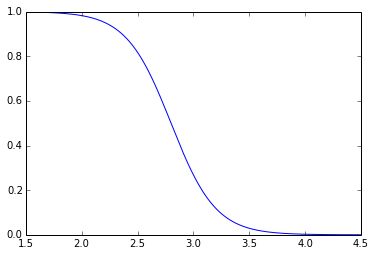From playing with that widget you can find a value that you're happy with. I chose a distribution that had the bats leaving about a quarter of the days. Remember, this is still in fake land, we are just generating some data to see how well pymc can infer stuff about our real model.

Let's actually do a logistic regression using the bat score and the simulated exits and see an upper bound on how good we can do with this modeling (for when we later throw in the twitter noise).

In :
wind_and_temp = np.vstack([wind_speeds, temperatures]).T
wind_temp_model = LogisticRegression()
wind_temp_model.fit(wind_and_temp, bat_exit_days)

Out:
LogisticRegression(C=1.0, class_weight=None, dual=False, fit_intercept=True,
intercept_scaling=1, penalty='l2', random_state=None, tol=0.0001)
In :
f1_score(wind_temp_model.predict(wind_and_temp), bat_exit_days)

Out:
0.85450346420323331
In :
grumpy_model = LogisticRegression()
grumpy_col = grumpy_bat_score.reshape((grumpy_bat_score.size,1))
grumpy_model.fit(grumpy_col, bat_exit_days)
f1_score(grumpy_model.predict(grumpy_col), bat_exit_days)

Out:
0.86374133949191689
In :
# Showing how the curves differ between the grumpy model and the generating model
x = np.linspace(0, 10, 100)
(grumpy_a, grumpy_b) = grumpy_model.raw_coef_.ravel()
y_1 = 1 / (1 + np.exp(-(x * grumpy_a + grumpy_b)))
plt.plot(x,y_1, label="modeled logistic curve")
y_2 = 1 / (1 + np.exp(-(x * widget_a + widget_b)))
plt.plot(x, y_2, label="actual logistic curve")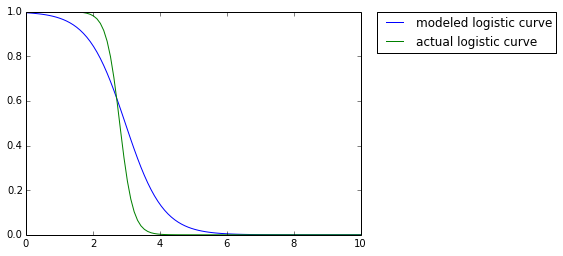The logistic curves look pretty good. When you increase the number of days that you sample, the modeled logistic curve sharpens (gets steeper in the middle) to match the actual logistic curve. Play with the notebook to see. It's cool.

Now let's say we didn't fabricate all this data, instead we have temperatures, wind speeds, and bat sightings. Logistic regression will give a model that fits the data well, but it will just be one set of parameters to the sigmoid function. In the first step in our analysis (we'll get to Twitter), we'll instead model the logistic regression parameters as unknowns and see what sort of distributions the data impose on them.

In :
def first_bat_model():
wind_effect = Normal('wind_effect', -5, 2)
temp_effect = Normal('temp_effect', -5, 2)
offset = Normal('offset', -5, 2)

@deterministic
def swarm_prob(t=temp_effect, w=wind_effect, c=offset):
return 1 / (1 + np.exp(-(temperatures*t + wind_speeds*w + c)))

bat_swarm = Bernoulli('bat_swarm', swarm_prob, value=bat_exit_days, observed=True)
return locals()

In :
M_1 = MCMC(first_bat_model())
M_1.sample(iter=1000000, burn=10000, thin=100)

 [-----------------100%-----------------] 1000000 of 1000000 complete in 142.4 sec
In :
Matplot.plot(M_1)

Plotting temp_effect
Plotting offset
Plotting wind_effect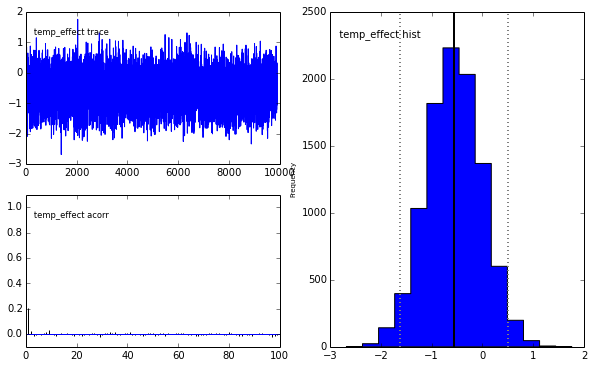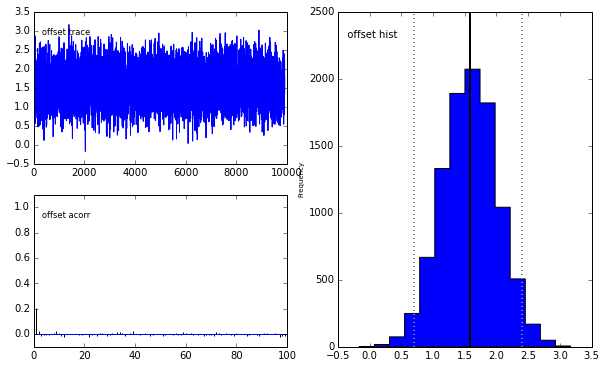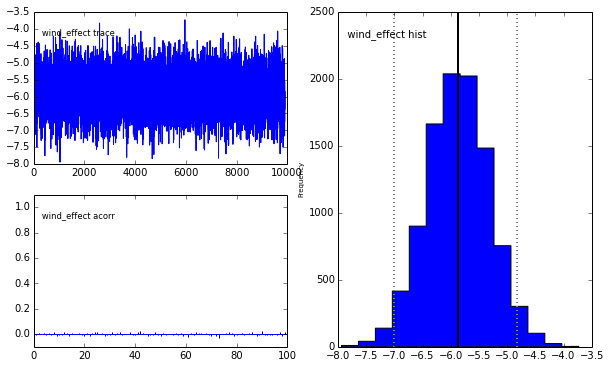In :
predictions = (M_1.swarm_prob.value > .5)

In :
f1_score(predictions, bat_exit_days)

Out:
0.8571428571428571

So this model does almost as well logistic regression above, even just taking the last sample from the sampling, and we get our snazzy distributions for the parameters. Should really be cross validating this stuff to see if we're actually being predictive and all though.

Now we are going to assume that we no longer have real knowledge of whether the bats leave or not. Instead we look at tweets that occur when the bats leave the bridge. If there are zero tweets it could mean that there were no bats. It could also mean that the crowd just doesn't have many people addicted to social media.

### Back to fake data generation land.¶

Looking over the last few days, it seems like when there are tweets about the bats showing up, there are usually 2,3 or 4. So given that bats occur, I think that a Poisson distribution with $\lambda = 3$ sounds fine.

In :
tweets = np.random.poisson(lam=3, size=NUM_DAYS) * bat_exit_days

In :
def second_bat_model():
wind_effect = Normal('wind_effect', -5, 1)
temp_effect = Normal('temp_effect', -5, 1)
offset = Normal('offset', 0, 1)

@deterministic
def swarm_prob(t=temp_effect, w=wind_effect, c=offset):
return 1 / (1 + np.exp(-(temperatures*t + wind_speeds*w + c)))

avg_tweets_when_sighted = TruncatedNormal('avg_tweets_when_sighted', 2, 1, a=.2, b=20)

# For some reason, just doing .asdtype(np.bool) was giving me things that didn't
# work with np.select down below
(tweets_bool, no_tweets_bool) = (tweets > 0, tweets == 0)

# Zero inflated poisson, as sometimes we get no tweets because of no bats,
# other times because people just didn't want to tweet
# Lifted from Fonnesbeck: https://gist.github.com/874808/bb06cbede6bd0840eabc15a516a89b0e38b4e135
@observed(dtype=int, plot=False)
def zippo(value=tweets, s=swarm_prob, l=avg_tweets_when_sighted):
prob_if_no_tweets = np.log((1-s) + s * np.exp(-l))
prob_if_tweets = np.log(s) + poisson_like(value, l)
v = np.select([no_tweets_bool, tweets_bool], [prob_if_no_tweets, prob_if_tweets])
return v.sum()

return locals()

In :
M_2 = MCMC(second_bat_model())
M_2.sample(iter=2000000, burn=20000, thin=100)

 [-----------------100%-----------------] 2000000 of 2000000 complete in 1103.9 sec
In :
Matplot.plot(M_2)

Plotting temp_effect
Plotting wind_effect
Plotting avg_tweets_when_sighted
Plotting offset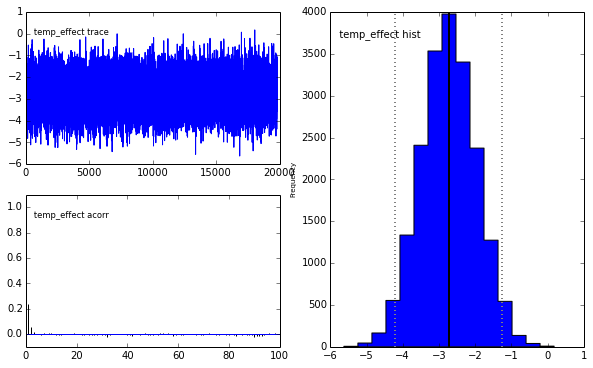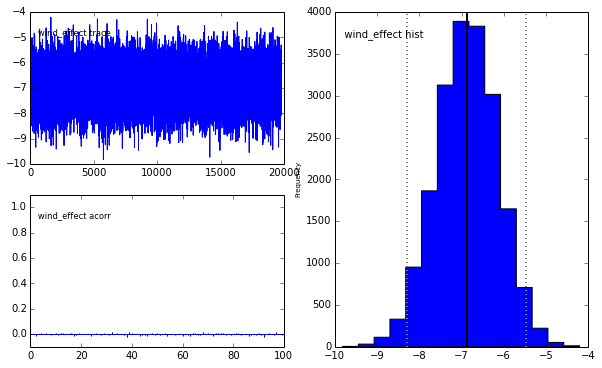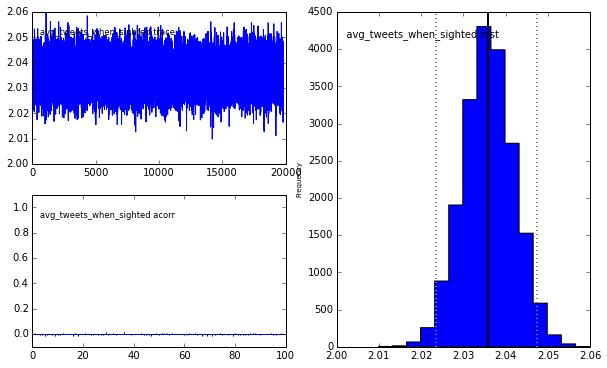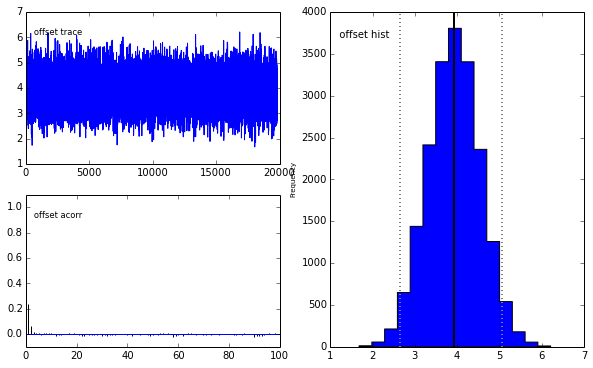In :
predictions = (M_2.swarm_prob.value > .5)

In :
(predictions == bat_exit_days).sum()

Out:
237
In :
f1_score(predictions, bat_exit_days)

Out:
0.85517241379310349

The f1 score is about as good as training the model on the actual bat exits as opposed to the data with the noise of Twitter included.

The next step here is to start scraping bat tweets until next years conference so that I have a good chance of seeing them! If you want to talk about any of this, e-mail me at [email protected] or tweet to @justinvf.

I will probably be creating a text classifier for the tweets so that adds another layer of complexity. Not only will the tweets be randomly dependent on the bats, but the classifier will be outputting a probability that the tweet is even about bats. It seems like it should fit naturally within the whole framework though.

A big huge thanks to @fonnesbeck for giving the pymc tutorial at this years SciPy and finding the bug in the zippo function (had commented out the decorator accidentally). Also, thanks to @Cmrn_DP for writing Probabilistic Programming and Bayesian Methods for Hackers